Latest SSC jobs   »   Target SSC CGL | 10,000+ Questions...

# Target SSC CGL | 10,000+ Questions | Quant Questions For SSC CGL : Day 133

This is the new year with new goals, new experiences and lots of exams to be scheduled soon. SSC CGL has recently released the exam dates so now it is time to gear up your preparations and try hard to get success. ADDA247 never fails to deliver something new and fruitful for you all. This time also we are providing you the best study plan as well as a study material. We are here going to prepare your Quantitative Aptitude section for the SSC CGL. In this article, we are providing you the details that how we are going to help you to clear the examination this year. We ADDA247 is going to provide you daily tests for all the subjects. The topic-wise quiz will be done from January till May. This will help you to get a deeper knowledge of all the topics and will prepare you thoroughly.

Q1. A sum of Rs. 1750 is divided into two parts such that the interests on the first part at 8% simple interest per annum and that on the other part at 6% simple interest per annum are equal. The interest on each part (in rupees) is
(a) 60
(b) 65
(c) 70
(d) 40

Q2. A sum of Rs. 1550 was lent partly at 5% and partly at 8% simple interest. The total interest received after 3 years is Rs. 300. The ratio of money lent at 5% to that at 8% is?
(a) 5 : 8
(b) 8 : 5
(c) 31 : 6
(d) 16 : 15

Q3. What sum of money will amount to Rs. 520 in 5 years and to Rs. 568 in 7 years at simple interest?
(a) Rs. 400
(b) Rs. 120
(c) Rs. 510
(d) Rs. 220

Q4. Rs. 500 was invested at 12% per annum simple interest and another certain sum of money invested at 10% per annum simple interest. If the total interest on both the sum after 4 years is Rs. 480, the latter sum of money is:
(a) Rs. 450
(b) Rs. 750
(c) Rs. 600
(d) Rs. 550

Q5. A money lender finds that due to fall in the annual rate of interest from 8% to 7¾%, his yearly income diminishes by Rs. 61.50. His capital is?
(a) Rs. 22400
(b) Rs. 23800
(c) Rs. 24600
(d) Rs. 26000

Q6. A certain sum of money amounts to Rs. 756 in 2 years and to Rs. 873 in 3½ years at a certain rate of simple interest. The rate of interest per annum is
(a) 10%
(b) 11%
(c) 12%
(d) 13%

Q7. What sum will amount to Rs. 7000 in 5 years at 3⅓% simple interest?
(a) Rs. 6300
(b) Rs. 6500
(c) Rs. 6000
(d) Rs. 5000

Q8. The simple interest on a certain sum for 8 months at 4% per annum is Rs. 129 less than the simple interest on the same sum for 15 months at 5% per annum. The sum is:
(a) Rs. 2,580
(b) Rs. 2400
(c) Rs. 2529
(d) Rs. 3600

Q9. A man took a loan from a bank at the rate of 12% per annum at simple interest. After 3 year he had to pay Rs. 5400 as interest only for that period. The principal amount borrowed by him was:
(a) Rs. 2000
(b) Rs. 10,000
(c) Rs. 20,000
(d) Rs. 15,000

Q10. Mohan lent some amount of money at 9% simple interest and an equal amount of money at 10% simple interest each for two years. If his total interest was Rs. 760, what amount was lent in each case?
(a) Rs. 1700
(b) Rs. 1800
(c) Rs. 1900
(d) Rs. 2000

Solutions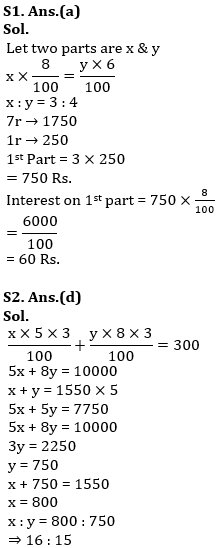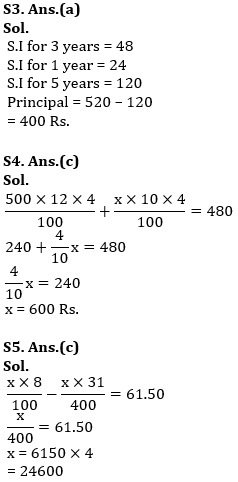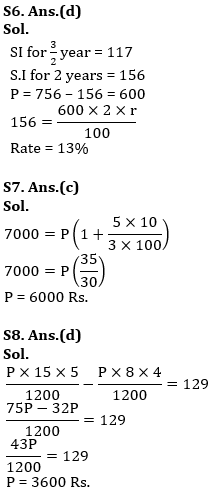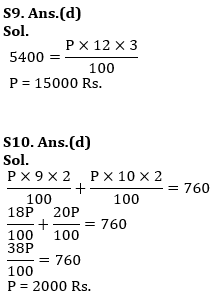In the last month i.e. in May daily we will provide you with a test of the previous years’ question papers, this will increase your confidence of solving the real exam and will make you familiar with the real-time exam.

Click here for best SSC CGL mock tests, video course, live batches, books or eBooks

Preparing for SSC Exams in 2020-21? Register now to get free study material

#### SSC CGL 2020 CAPSULE General Awareness And General Science: Free PDF | Download Now

Click here for best SSC CGL mock tests, video course, live batches, books or eBooks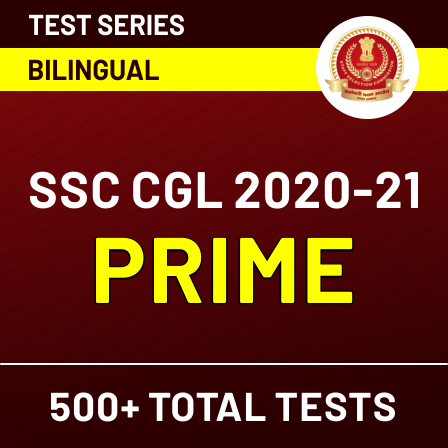#### Congratulations!General Awareness & Science Capsule PDF